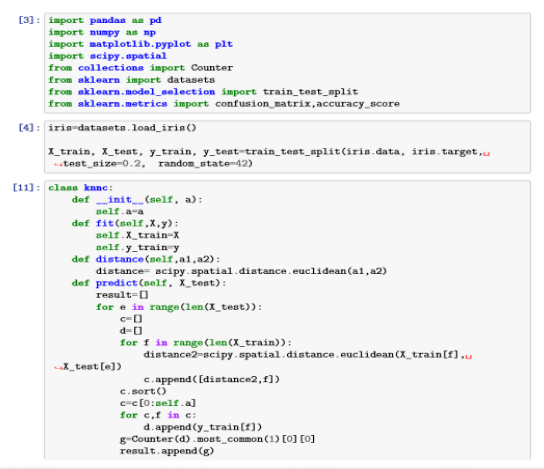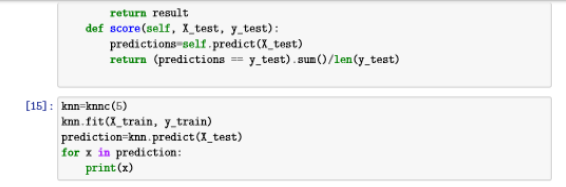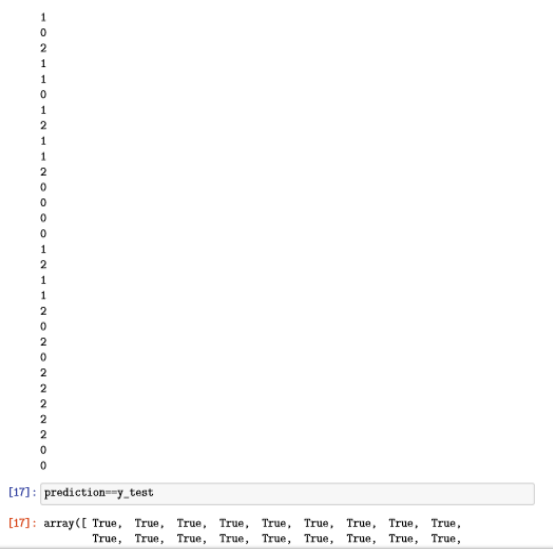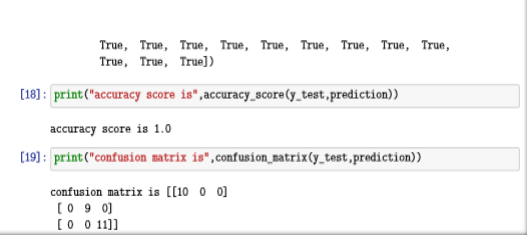# Python Learning Week 9 (Solution)

## KNN Classifier In Artificial Learning

Implement KNN algorithm yourself in python for Iris Dataset without using built-in KNN classifier library.

2. Split dataset into test and train sets
3. Perform KNN algorithm to make predictions for k=5
4. Compute accuracy and confusion matrix
CODE:What we studied in Week 9:
In this week we studied the Implementation of K-Nearest Neighbor Classification Model. This machine learning algorithm is one of the types of machine learning
algorithm which is dependent on labelled input data in order to learn a function
which is capable of producing an output whenever a new unlabeled data is given
as input. K-NN is widely used in real life scenarios, as it is non-parametric which
means that it does not make any underlying assumptions about the distributions
of data. Advantage of KNN is that it is easy to use and it provides quick calculation time. We also implemented this algorithm in python as well. This is all we have studied in this week.